# linearArray

Create linear antenna array

## Description

The `linearArray` class creates a linear antenna array in the X-Y plane. By default, the linear array is a two-element dipole array. The dipoles are center fed. Each dipole resonates at 70 MHz when isolated.## Creation

### Syntax

``array = linearArray``
``array = linearArray(Name,Value)``

### Description

example

``` `array = linearArray` creates a linear antenna array in the X-Y plane. ```

example

``` `array = linearArray(Name,Value)` class to create a linear antenna array, with additional properties specified by one, or more name-value pair arguments. `Name` is the property name and `Value` is the corresponding value. You can specify several name-value pair arguments in any order as `Name1`, `Value1`,`...`, `NameN`, `ValueN`. Properties not specified retain their default values.```

### Output Arguments

expand all

Linear array, returned as an `linearArray` object.

## Properties

expand all

Individual antenna elements or array elements, specified as an antenna or array object.

Example: `'Element',monopole`

Number of antenna elements in array, specified as a scalar.

Example: `'NumElements'`,4

Spacing between antenna elements, specified as a scalar or vector in meters. By default, the dipole elements are spaced `2 m` apart.

Example: `'ElementSpacing',3`

Data Types: `double`

Excitation amplitude of antenna elements, specified as a scalar or vector. Set the property value to `0 ` to model dead elements. This value corresponds to the excitation voltages for the elements in the array.

Example: `'AmplitudeTaper',3`

Data Types: `double`

Phase shift for antenna elements, specified as a scalar or vector in degrees. This value corresponds to the excitation voltages for the elements in the array.

Example: `'PhaseShift',[3 3 0 0]`

Data Types: `double`

Tilt angle of the array specified as a scalar or vector with each element unit in degrees. For more information, see Rotate Antennas and Arrays.

Example: `'Tilt',90`,

Example: `'Tilt',[90 90]`,`'TiltAxis',[0 1 0;0 1 1]` tilts the array at 90 degrees about the two axes, defined by vectors.

Data Types: `double`

Tilt axis of the array, specified as:

• Three-element vectors of Cartesian coordinates in meters. In this case, each vector starts at the origin and lies along the specified points on the X-, Y-, and Z-axes.

• Two points in space, each specified as three-element vectors of Cartesian coordinates. In this case, the array rotates around the line joining the two points in space.

• A string input describing simple rotations around one of the principal axes, 'X', 'Y', or 'Z'.

Example: `'TiltAxis',[0 1 0]`

Example: `'TiltAxis',[0 0 0;0 1 0]`

Example: `array.TiltAxis = 'Z'`

Data Types: `double`

## Object Functions

 `show` Display antenna or array structure; display shape as filled patch `info` Display information about antenna or array `beamwidth` Beamwidth of antenna `charge` Charge distribution on metal or dielectric antenna or array surface `correlation` Correlation coefficient between two antennas in array `current` Current distribution on metal or dielectric antenna or array surface `design` Design prototype antenna or arrays for resonance around specified frequency `efficiency` Radiation efficiency of antenna `EHfields` Electric and magnetic fields of antennas; Embedded electric and magnetic fields of antenna element in arrays `impedance` Input impedance of antenna; scan impedance of array `layout` Display array or PCB stack layout `mesh` Mesh properties of metal or dielectric antenna or array structure `optimize` Optimize antenna or array using SADEA optimizer `pattern` Radiation pattern and phase of antenna or array; Embedded pattern of antenna element in array `patternAzimuth` Azimuth pattern of antenna or array `patternElevation` Elevation pattern of antenna or array `rcs` Calculate and plot radar cross section (RCS) of platform, antenna, or array `returnLoss` Return loss of antenna; scan return loss of array `sparameters` Calculate S-parameter for antenna and antenna array objects

## Examples

collapse all

Create a linear array of four dipoles and plot the layout of the array.

```la = linearArray; la.NumElements = 4; layout(la);```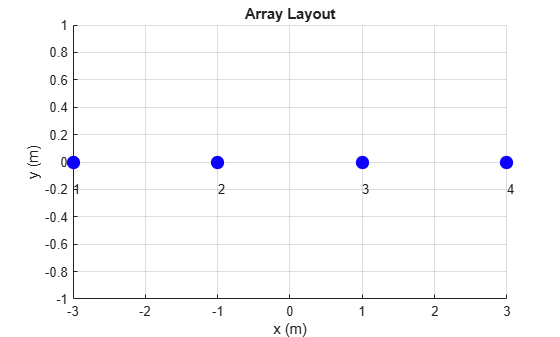Plot the radiation pattern of a four element linear array of dipoles at a frequency 70MHz.

```la = linearArray('NumElements',4); pattern(la,70e6);```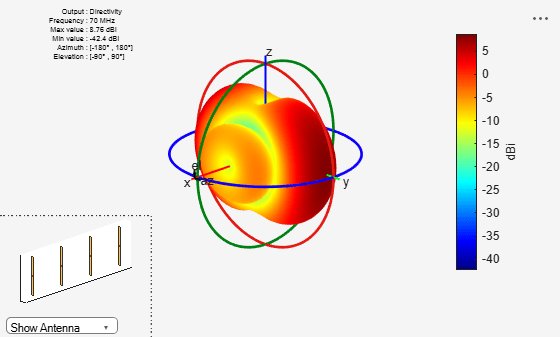Create a linear array of two monopoles.

```m1 = monopole; m2 = monopole('Height',0.5); mla = linearArray```
```mla = linearArray with properties: Element: [1x1 dipole] NumElements: 2 ElementSpacing: 2 AmplitudeTaper: 1 PhaseShift: 0 Tilt: 0 TiltAxis: [1 0 0] ```
```mla.Element = [m1,m2]; show(mla);```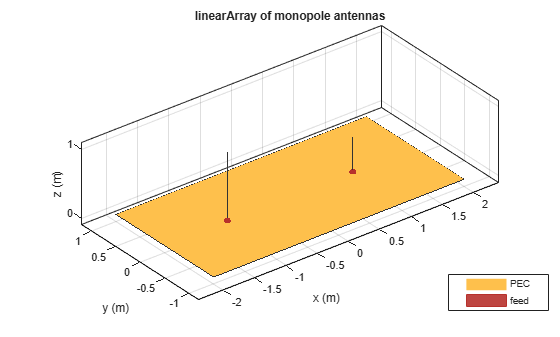Create an array of discones with element spacing of 3 m.

```la = linearArray('Element',discone); la.ElementSpacing = 3; show(la)```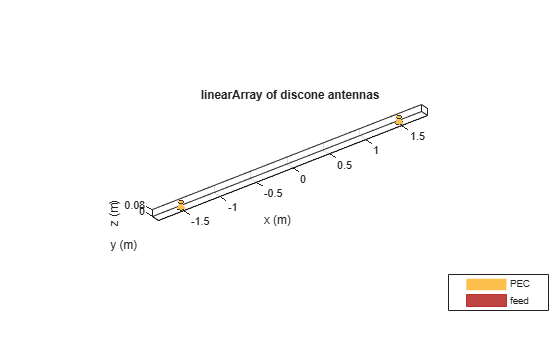Create a rectangular of the linear array.

`ra = rectangularArray("Element",la)`
```ra = rectangularArray with properties: Element: [1x1 linearArray] Size: [2 2] RowSpacing: 2 ColumnSpacing: 2 Lattice: 'Rectangular' AmplitudeTaper: 1 PhaseShift: 0 Tilt: 0 TiltAxis: [1 0 0] ```
`show(ra)`Create a linear array and plot the pattern.

```la=linearArray('Element',linearArray('ElementSpacing',1)); show(la)```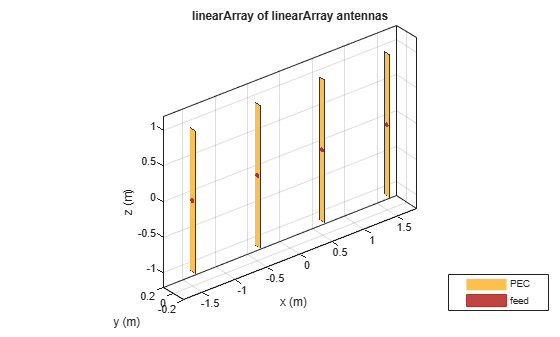`pattern(la,70e6); `Balanis, C.A. Antenna Theory. Analysis and Design, 3rd Ed. New York: Wiley, 2005.